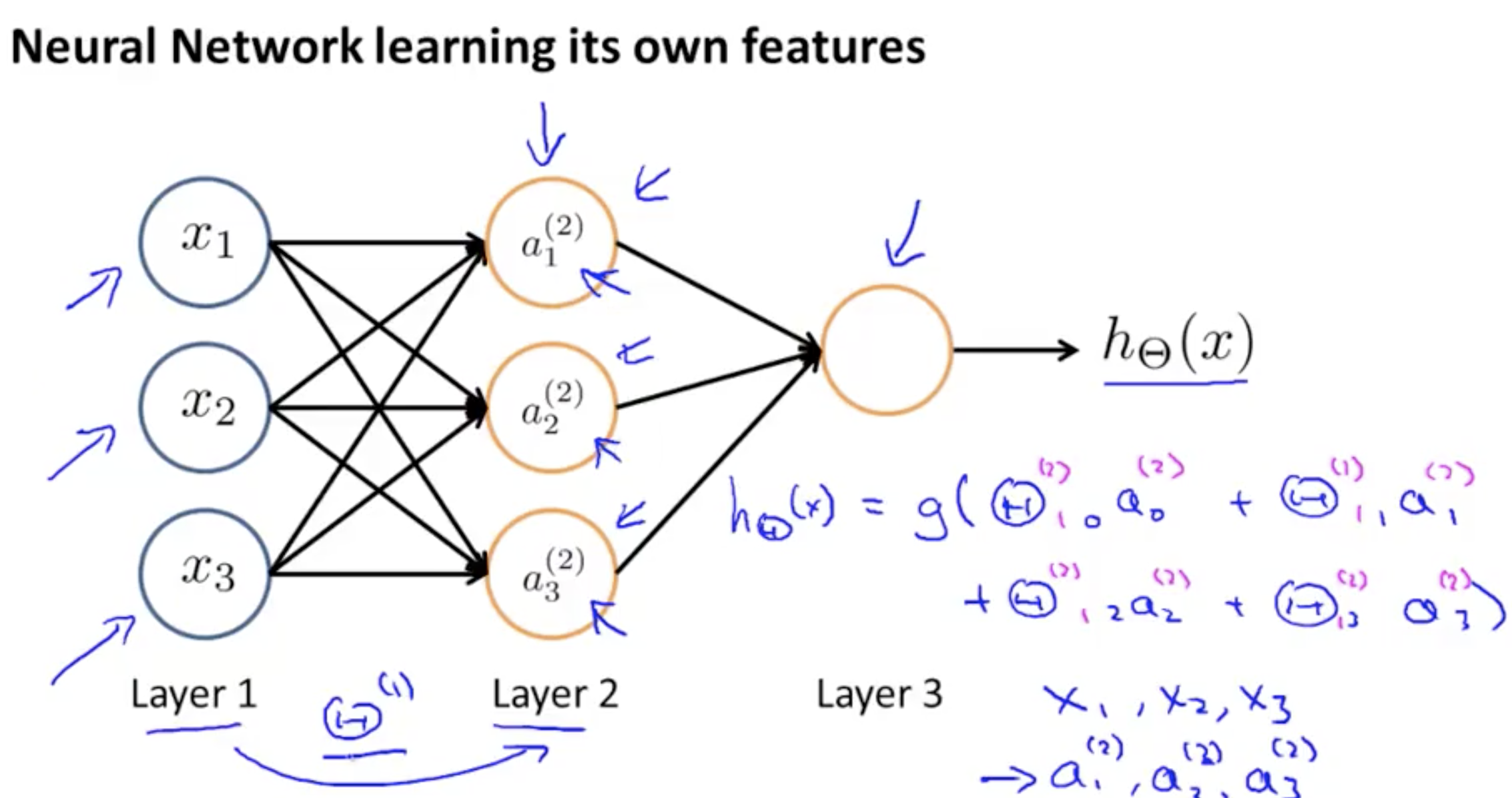# [L4] Neural Networks. Hypothesis and Representation.¶

Neural networks have been around for decades. But it hasn't been until recently, with the rise of big data and the availability of ever increasing computation power, that we have really started to see a lot of exciting progress in this branch of machine learning.

The most ground breaking advances in the field of machine learning over the past decade, from computer vision to NLP, can be attributed to the rise of neural networks, and in particular deep learning.

Neural networks roughly model the "gating" functionality of biological neurons in the brain. In machine learning, we represent these neurons as "activation" units. In a computer neural network, these activation units are arranged in a number of different "layers". At its core, each activation unit in the network takes an input, runs that input through some sort of non-linear activation function (such as a sigmoid or ReLU), and produces an output value which is then passed through the next layer in the network.

By arranging large amounts of these layers and activation units together, we can construct a neural network that can take some sort of labelled data as it's input, and learn to make relevant predictions.

Adding more layers to a neural network often results in more accurate predictions. The term deep learning comes from neural networks that have multiple layers of activation units.

The following post is a theoretical introduction to neural nets, and is a set of summarised notes from lesson 4 of Andrew Ng's Machine Learning Standford course on Coursera. We start by learning how we represent neural networks in terms of math and code. We cover the structure of a basic neural net, how a hypothesis function looks like in neural net, and also start to represent some of the theory and bring that into some Matlab code.

## Representation: Inputs (x) and Activation units (a)¶

### A single model of a neuron¶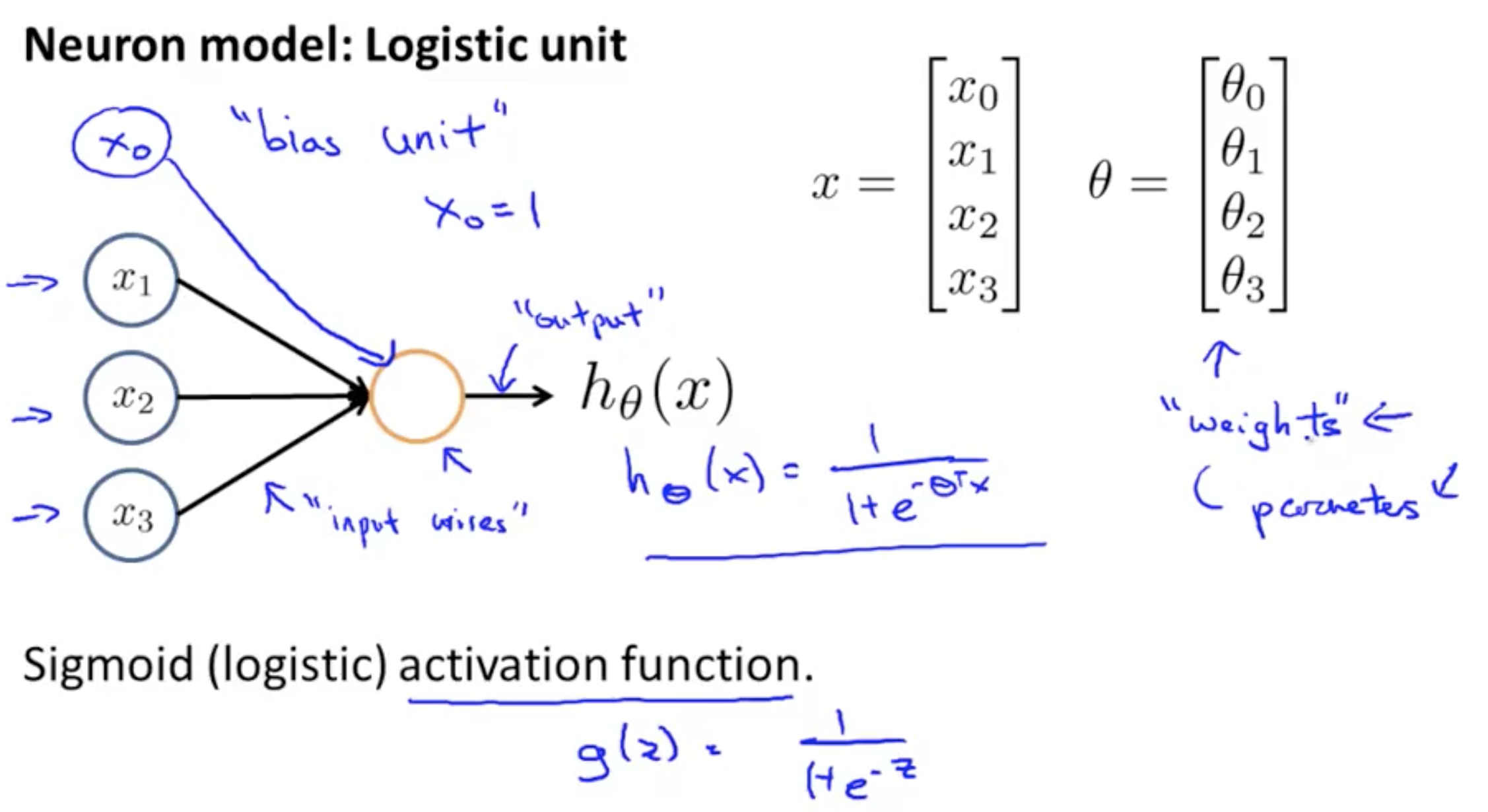A simplistic representation of what the above looks like this.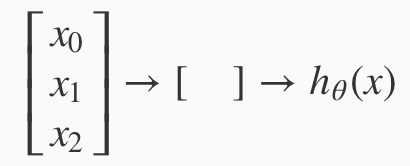### Neural network¶

A neural network is composed of many neurons. Neurons are arranged into linear layers, but have non-linear activations. The internal layers of a neural network are called the "hidden" layers.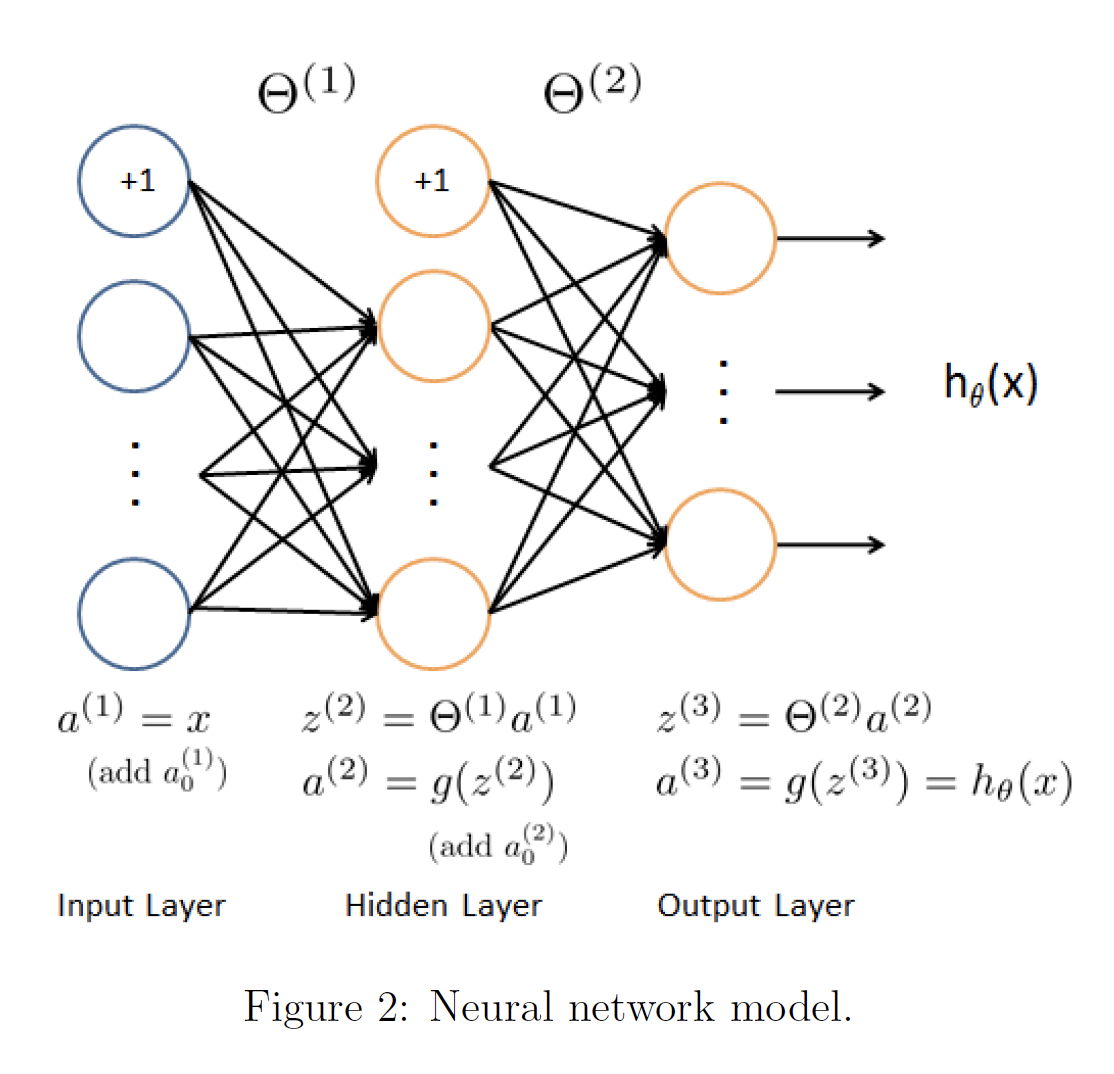Now if we had one hidden layer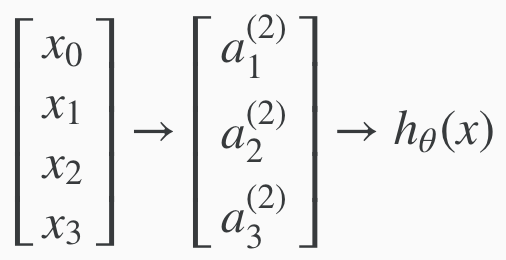For the first activation unit, its value can be obtained by using the sigmoid function against the sum of its weights and inputs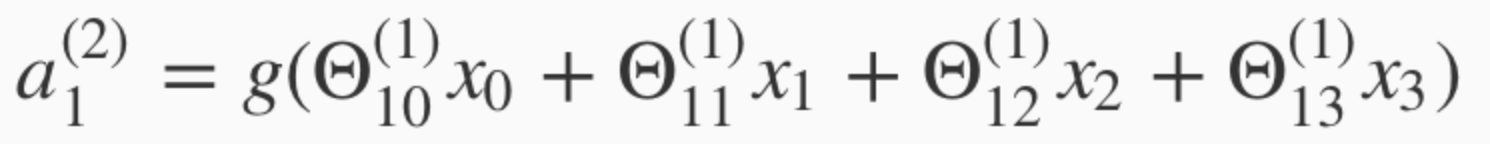Note that now for that single activation unit (a), we can collect the thetas into a vector, and X features into a vector.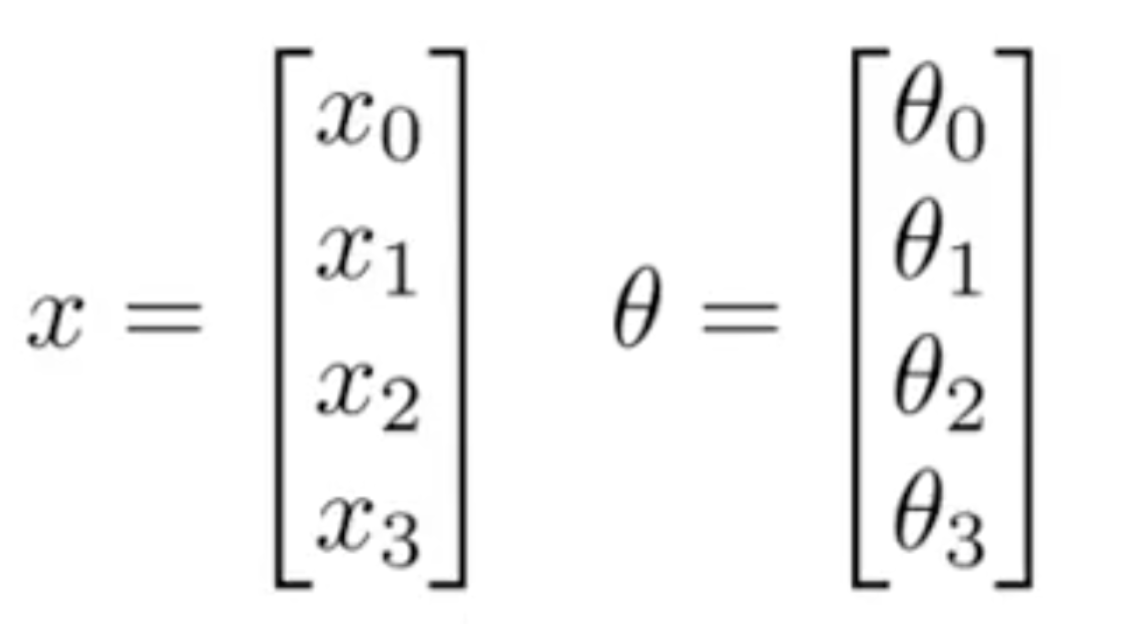All activation units (a) values can then be obtained like so: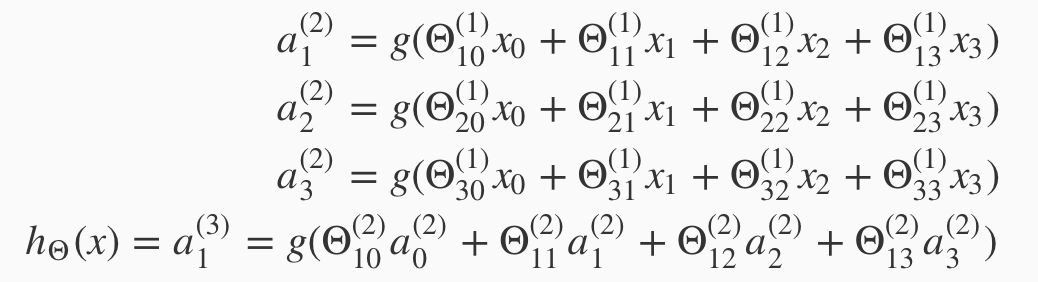The dimensions of these matrices of weights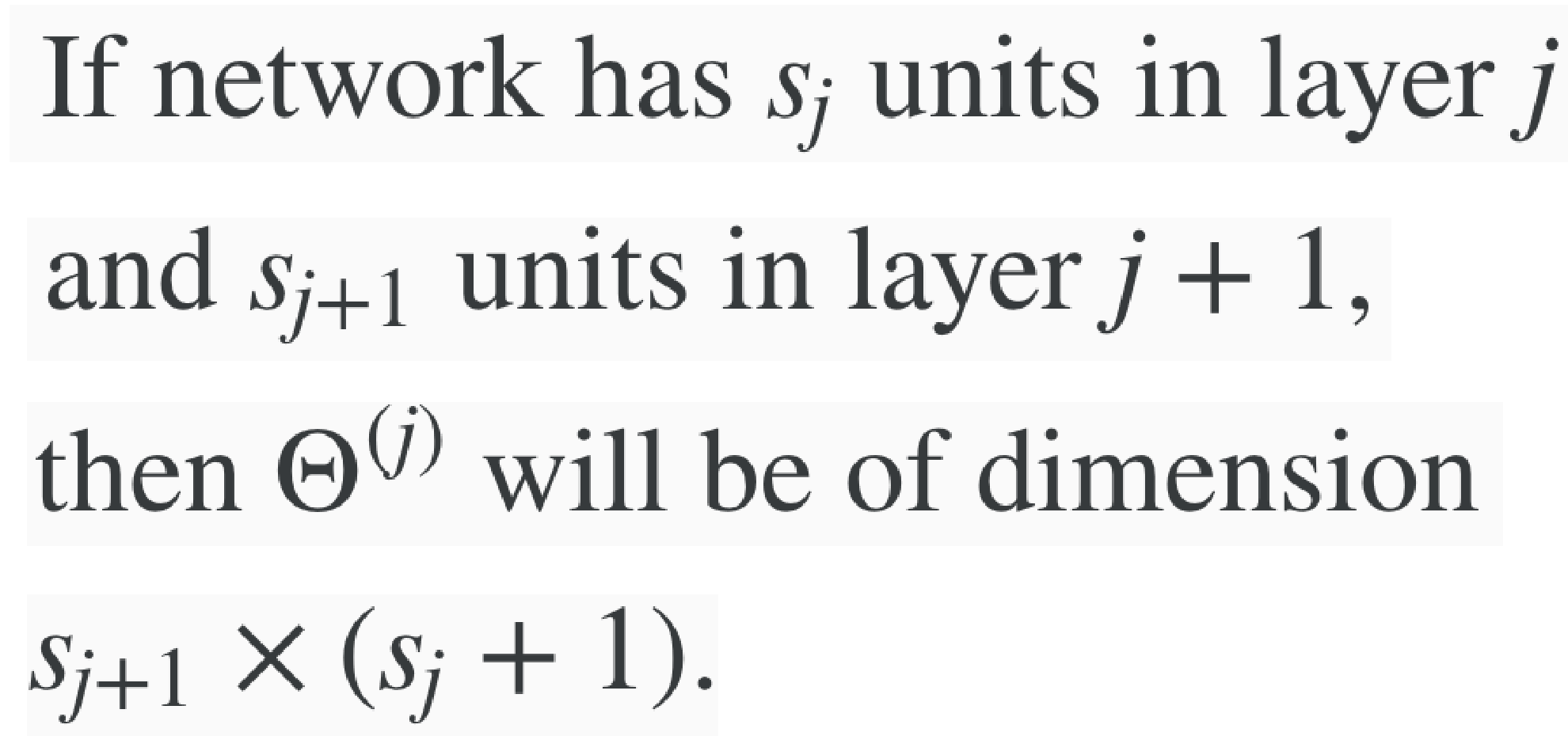The +1 comes from the addition in Theta^j of the "bias nodes," x0 â€‹ and Theta0^j

Note: Knowing the dimensions of the Theta matrix is important. When you are using matrix multiplication with Neural Nets, it will be useful to know the order in which to apply the Theta matrix in

1. The number of rows of the Theta matrices correspond to the number of "target" activation units.

2. The number of columns of the Theta matrices correspond to the number of "source" input units

## Hypothesis: In terms of theta and X¶

Activation of unit i, in layer j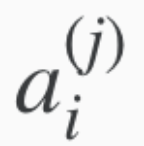Notation for activation units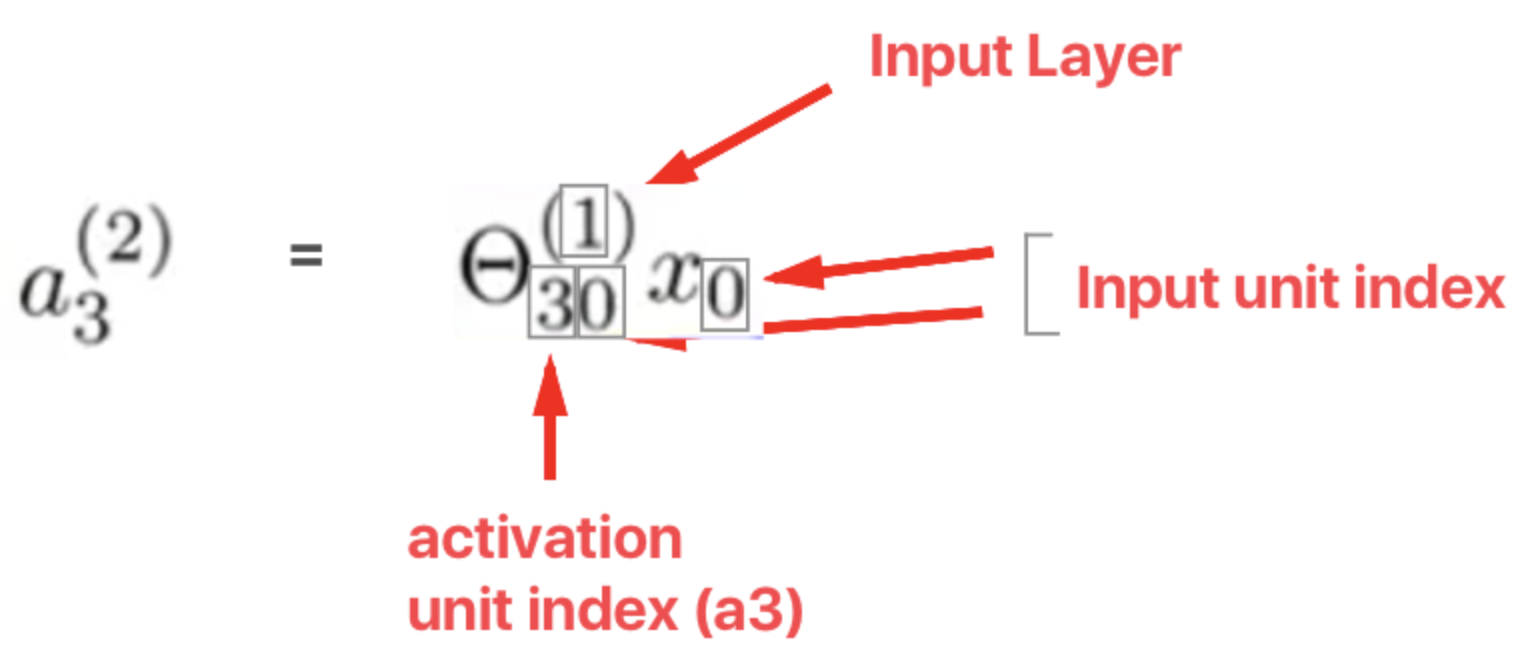Each layer gets its own matrix of weights. Matrix of weights controlling function mapping from layer j to layer j+1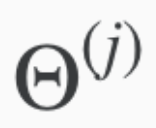Reiterating how to obtain values of the activation units.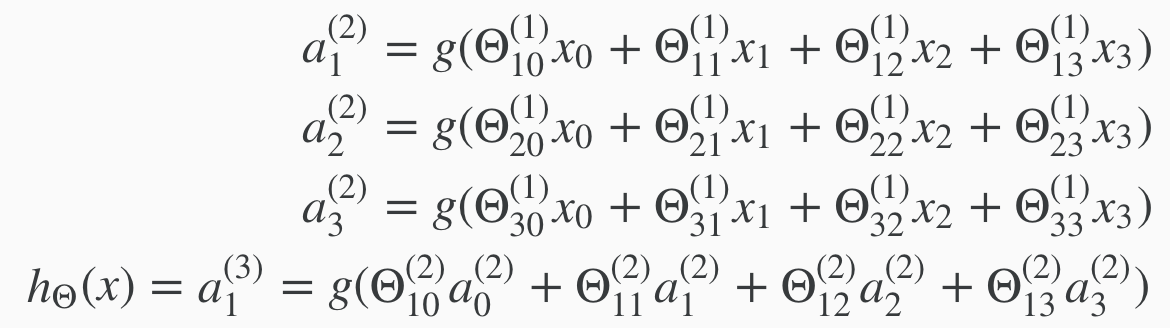1. Now we assign a new value "z" to the inputs and theta weights.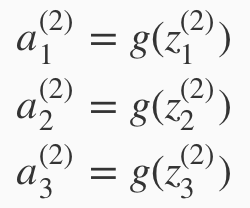2. In other words, for layer j=2 and node k, the variable z will be: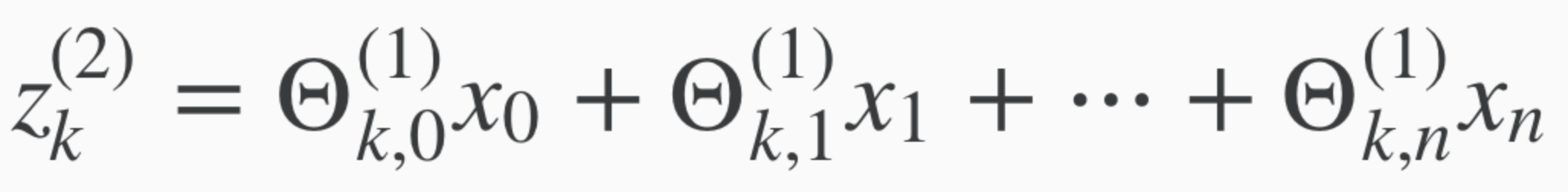3. Turning x and z of j into vectors gives us this.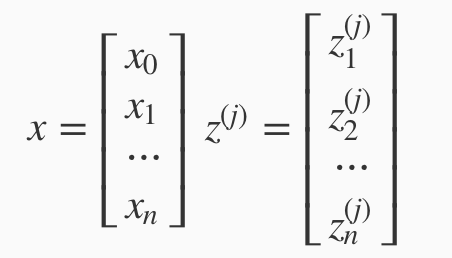4. Now we can express z, generally for layer j as: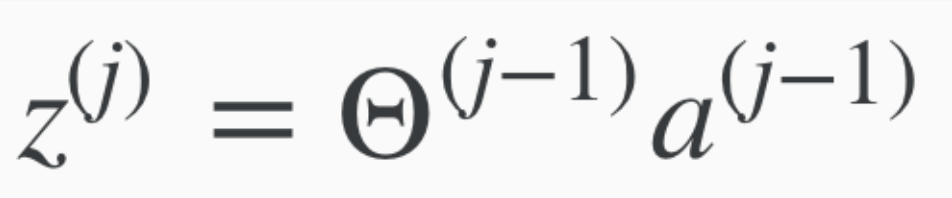5. Therefore we can also express z j+1 as this.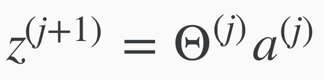6. Therefore, for activation unit a of j, we can apply the sigmoid function g "element-wise" to the matrix Z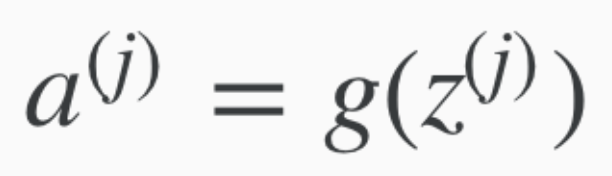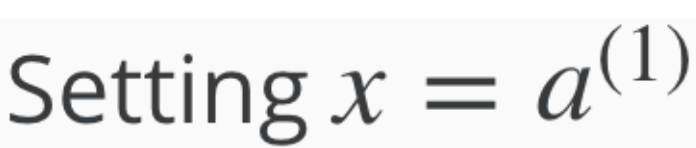7. Which gives us the hypothesis: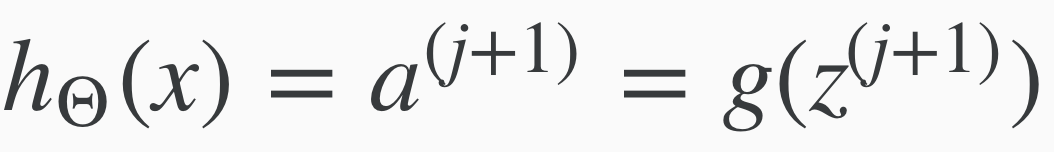Example, to compute the a(superscript 2) layer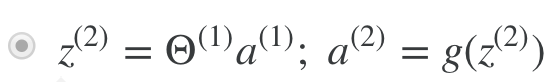## Vectorising¶

See the Coursera summary for a step by step run through of how to vectorise a Neural Net. https://www.coursera.org/learn/machine-learning/supplement/YlEVx/model-representation-ii

## Forward Propagation.¶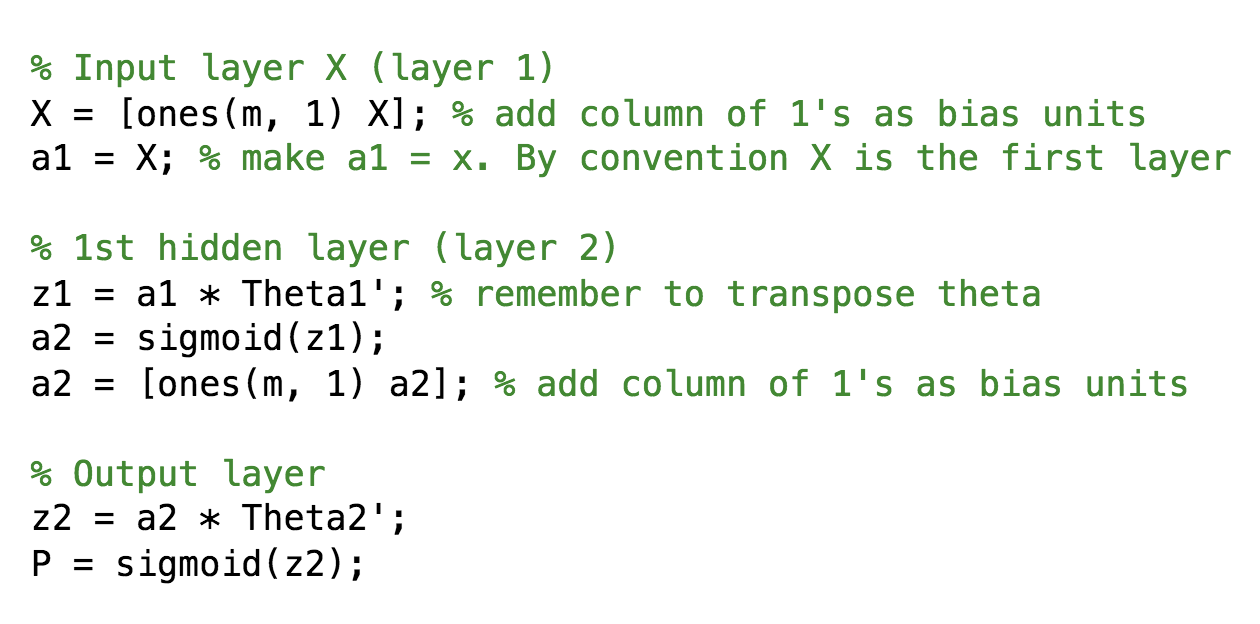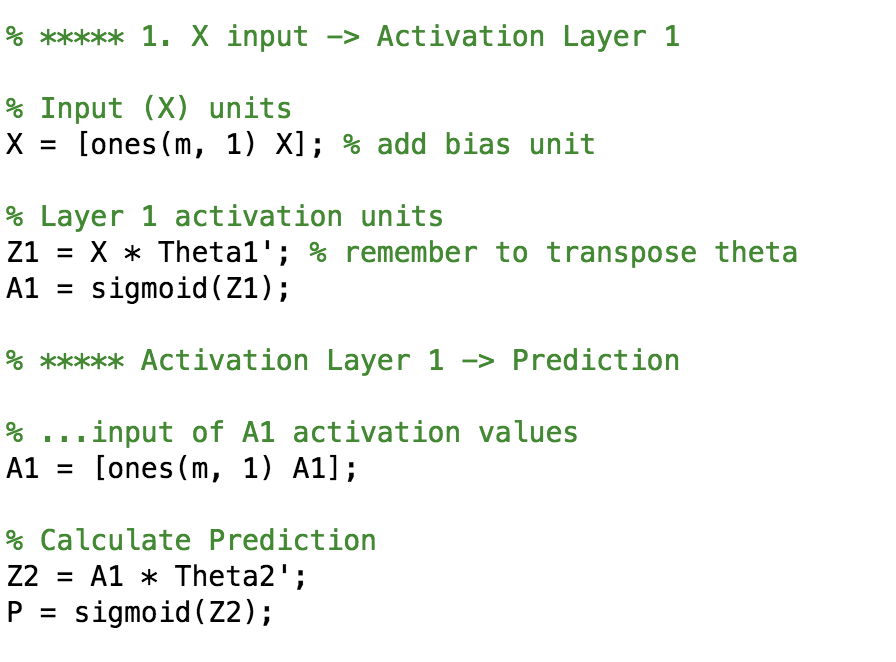The above code:

1. X is a matrix of input features. Its rows correspond to the number of training examples. Its columns to the number of features. It has a dimension of: m example rows x n feature columns
2. We add a column of 1s to X as bias units. (Important: Add bias units 1st before you transpose)
3. By convention we make a1 = X, as X is the first layer.
4. Then we calculate z by a1 * Theta1 (transpose). The transpose is important so that the inner product of the a1 matrix and the Theta1 matrix are the same to allow for matrix multiplication.
5. To get a2, we then we apply the sigmoid function to z element-wise. sigmoid(z)
6. Now with a2, we repeat the process to get the final out put layer.
7. We add bias units to a2, then apply the sigmoid function to its element z also.

## Theta¶

1. Theta1 and Theta2 are pre-trained matrices of theta values for a single layer neural network. Theta1 are the weights applied to the feature input matrix X. Theta2 are the weights applied to get the output units.
• The number of rows of the Theta matrices correspond to the number of "target" activation units.
• The number of columns of the Theta matrices correspond to the number of "source" input units (including the bias unit)
• So Theta1 is num "target" activation units x num "source" input units (including the bias unit)
2. Recall that X has m example rows x n feature columns
3. Feature columns of X is the inner component of its matrix. This needs to match with the inner component of Theta
4. To do this, you can transpose Theta so that its columns which correspond to the number of "source" inputs, is now the rows of Theta after a trans pose.
5. So now: X (m x n inputs) * Theta1' (n inputs x activation units)

## Prediction¶

Calculating training set accuracy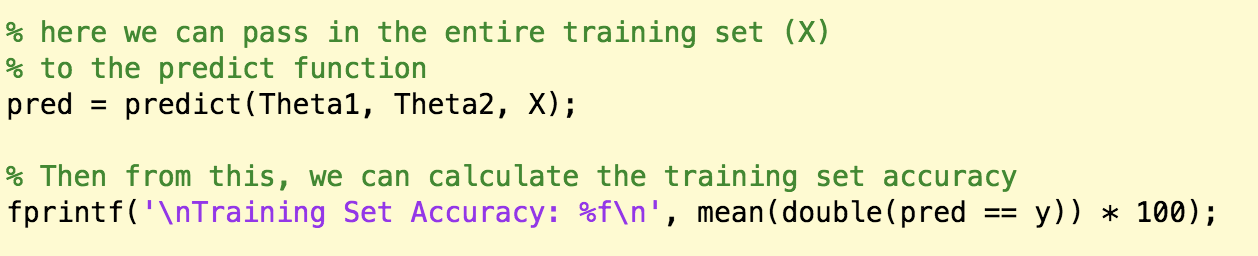Predict from passing in a single training example.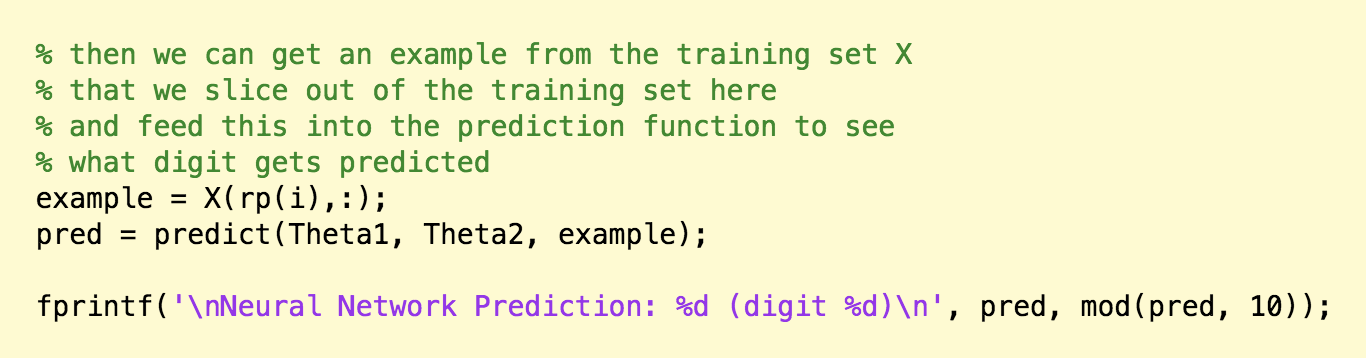Neural nets, learning their own features: So it's as if the neural network, instead of being constrained to feed the features x1, x2, x3 to logistic regression. It gets to learn its own features, a1, a2, a3, to feed into the logistic regression and as you can imagine depending on what parameters it chooses for theta 1. You can learn some pretty interesting and complex features and therefore 8:43 you can end up with a better hypotheses than if you were constrained to use the raw features x1, x2 or x3 or if you will constrain to say choose the polynomial terms, you know, x1, x2, x3, and so on